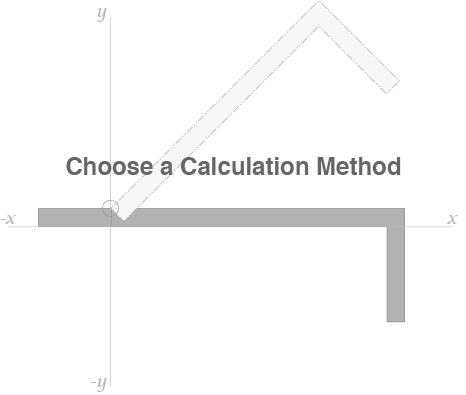Torque Hinge CalculatorUniform Weight Distribution

Calculate based on weight density (specific gravity)

Select Material
Flap Length (L)   mm
Flap Width   mm
Flap Thickness (T)   mm
X Distance
mm
Y Distance
mm
Flap Weight
kg

Uniform Weight Distribution

Measure based on flap length and weight

Flap Length (L) mm
Flap Weight   kg
Flap Calculated Weight
0
kg
L Value
0
mm
Minimum Torque Required
Fill in the values and this will automatically calculate
0
N⋅m
0
kgf·cm

*This calculation is a general estimate only. Depending on the materials used, the resulting weight may be different.